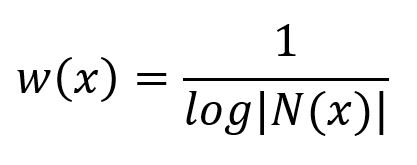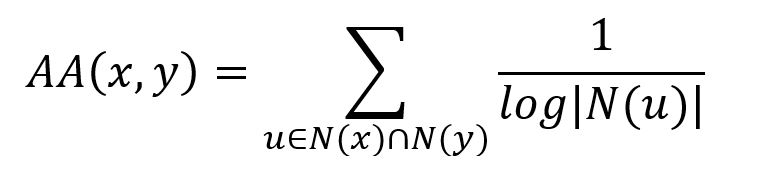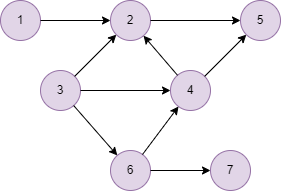# Change Nickname

Current Nickname:

Certifications

Certificate Issued at Valid until Serial No. File
Serial No. Valid until

Not having one? Apply now! >>>

Invoice

ProductName CreateTime ID Price File
ProductName CreateTime ID Price File

## Overview

Adamic-Adar index is a node similarity metric defined based on the structured information of the internet, this is how it differs from Jaccard similarity (semi-structured information). AA index uses the weights of the common neighbor nodes of two nodes as the similarity of the two nodes, it was proposed by Lada A. Adamic and Eytan Adar in 2003, related materials are:

## Basic Concept

### Node Weight

In AA index, the weight of a node `x` is defined as the reciprocal of the logarithm based on 10 of the size of the node's neighbor set `N(x)`:Weight of the yellow node in the graph below is: `1/(log4) = 1.6610`, weight of the green node is: `1/(log3) = 2.0959`.### AA Index

AA index uses the sum of weights of the common neighbors of two nodes to determine their closeness. It is calculated by the following formula:where `N(x)` and `N(y)` are neighbor sets of `x` and `y` respectively, `u` is the common neighbor of `x` and `y`. The larger the value of `AA(x,y)` is, the closer the two nodes are, value of 0 indicates that the two nodes are not close.

Still taking the previous graph as an example, AA index of the blue and red nodes is the sum of weights of the yellow and green nodes, which is `1/(log4) + 1/(log3) = 3.7569`.

## Special Case

### Lonely Node, Disconnected Graph

There is no edge between lonely node and any other nodes in the graph, the algorithm does not calculate AA index between lonely node and any other node, nor does it calculate AA index between two nodes in different connected components.

### Self-loop Edge

The algorithm ignores all self-loop edges when calculating neighbor nodes.

### Directed Edge

For directed edges, the algorithm ignores the direction of edges but calculates them as undirected edges.

## Results and Statistics

Take the graph below as an example, run the algorithm in the graph:Algorithm results: Calculate AA index for node 3 and other nodes, return `node1`, `node2` and `num`

node1 node2 num
3 1 1.660964047443681
3 2 1.660964047443681
3 4 3.7568673217330657
3 5 3.321928094887362
3 6 1.660964047443681
3 7 2.095903274289385

Algorithm statistics: N/A

## Command and Configuration

• Command: `algo(topological_link_prediction)`
• Configurations for the parameter `params()`:
Name
Type
Default
Specification Description
ids / uuids []`_id` / []`_uuid` / Mandatory IDs or UUIDs of the first set of nodes to be calculated, only need to configure one of them; every node in ids/uuids will be paired with every node in ids2/uuids2 for calculation
ids2 / uuids2 []`_id` / []`_uuid` / Mandatory IDs or UUIDs of the second set of nodes to be calculated, only need to configure one of them; every node in ids/uuids will be paired with every node in ids2/uuids2 for calculation
type string Adamic_Adar Adamic_Adar / Common_Neighbors / Preferential_Attachment / Resource_Allocation / Total_Neighbors Measurement of the closeness of the node pair; Adamic_Adar means to calculate AA index, Common_Neighbors means to calculate the number of common neighbors, Preferential_Attachment means to calculate the score of preferential attachment, Resource_Allocation means to calculate the score of resource allocation, Total_Neighbors means to calculate the number of total neighbors
limit int -1 >=-1 Number of results to return; return all results if sets to -1 or not set

## Algorithm Execution

#### 1. File Writeback

Configuration Data in Each Row
filename `node1`,`node2`,`num`

Example: Calculate AA index of node UUID = 3 and all other nodes, write the algorithm results back to file named aa

``````algo(topological_link_prediction).params({
uuids: ,
uuids2: [1,2,4,5,6,7]
}).write({
file:{
filename: "aa"
}
})
``````

#### 2. Property Writeback

Not supported by this algorithm.

#### 3. Statistics Writeback

This algorithm has no statistics.

### Direct Return

Alias Ordinal Type
Description
Column Name
0 []perNodePair Closeness of node pair `node1`, `node2`, `num`

Example: Calculate AA index of node UUID = 3 and UUID = 4, define algorithm results as alias named similarity and return the results

``````algo(topological_link_prediction).params({
uuids: ,
uuids2: ,
}) as similarity
return similarity
``````

### Streaming Return

Alias Ordinal Type
Description
Column Name
0 []perNodePair Closeness of node pair `node1`, `node2`, `num`

Example: Calculate AA index of node UUID = 1 and UUID = 5,6,7, return the results in the descending closeness score

``````algo(topological_link_prediction).params({
uuids: ,
uuids2: [5,6,7],